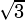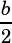Hi, I had some trouble with question #5 on p.242 of the PWN the SAT Math Guide book.
I read the solution and just cannot figure out how to get the value of h, (b^3)/2.
Could you explain the steps on how to get the value of h? Do I have to use Pythagorean theorem or is it just solving for “h” in the triangle area formula?

Hi, thanks for the question. The key here is that you’re dealing with equilateral triangles (a regular hexagon breaks up into six equilateral triangles).

Equilateral triangles have angles of 60°, so when you drop a segment from one vertex to the midpoint of the opposite side, you break the equilateral triangle into two 30°-60°-90° triangles. And those have special side length ratios, so they save us the trouble of needing to do the Pythagorean theorem: if the short leg is 1, then the long leg isand the hypotenuse is 2.

In the solution, I’m using all that to say that if the side of the original equilateral triangle is, then the hypotenuse of the 30°-60°-90° is, which means the short leg will be, and the long leg will be.